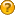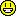###Author Topic: [Scripts] Random Reward Script  (Read 1248 times)

0 Members and 1 Guest are viewing this topic.

#### Forgeplex##### Random Reward Script
« on: April 06, 2018, 06:04:33 PM »
Does anybody know how to make this?

#### Lunar##### Re: Random Reward Script
« Reply #1 on: April 07, 2018, 12:37:01 PM »

var [num] = [rand:2]

If
IsVar [num] [=] 
Then
ElseIf
IsVar [num] [=] 
Then
ElseIf
IsVar [num] [=] 
Then
Else
EndIf

If this is what you mean it just gets a random number between 0-2, and then gives a certain item for each number

#### Craig##### Re: Random Reward Script
« Reply #2 on: April 08, 2018, 02:59:45 AM »

var [num] = [rand:2]

If
IsVar [num] [=] 
Then
ElseIf
IsVar [num] [=] 
Then
ElseIf
IsVar [num] [=] 
Then
Else
EndIf

If this is what you mean it just gets a random number between 0-2, and then gives a certain item for each number

A slightly more streamlined version. Since if num is not 0 and not 1 we know it must be 2 so the last ElseIf is unnecessary.

var [num] = [rand:2]
If
IsVar [num] [=] 
Then
ElseIf
IsVar [num] [=] 
Then
Else
EndIf

#### Forgeplex##### Re: Random Reward Script
« Reply #3 on: April 15, 2018, 03:38:54 PM »

var [num] = [rand:2]

If
IsVar [num] [=] 
Then
ElseIf
IsVar [num] [=] 
Then
ElseIf
IsVar [num] [=] 
Then
Else
EndIf

If this is what you mean it just gets a random number between 0-2, and then gives a certain item for each number

A slightly more streamlined version. Since if num is not 0 and not 1 we know it must be 2 so the last ElseIf is unnecessary.

var [num] = [rand:2]
If
IsVar [num] [=] 
Then
Thanks to Craig and Lunar!